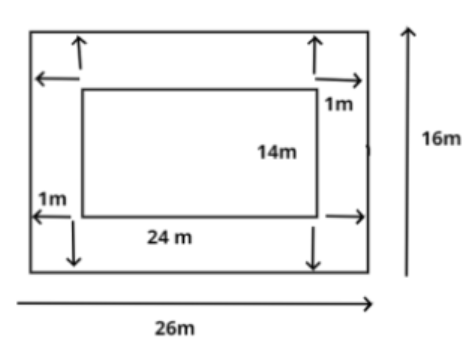Courses
Courses for Kids
Free study material
Offline Centres
MoreLast updated date: 26th Nov 2023
Total views: 382.2k
Views today: 3.82k

# A garden is 24 m long and 14 m wide. There is a path 1 m wide outside the garden along its side. If the path is to be constructed with square marble tiles 20 cm $\times$ 20 cm, find the number of tiles required to cover the path?$(a){\text{ }}1800 \\ (a){\text{ }}200 \\ (a){\text{ }}2000 \\ (a){\text{ }}2150 \\$Verified
382.2k+ views
Hint: The garden of given dimensions forms a rectangle of length 24m and breadth 14m. The 1 meter wide path to the exterior of the garden will eventually increase its length and breadth by 2m, 1m at top and 1 m at bottom in case of breadth, 1m more at left and 1 m more at right in case of length. It will be clearer after the pictorial representation of the dimensions.Now the given dimension of garden is $24m \times 14m$.That is length = 24m and breadth = 14m
As we know that the area of rectangle is given as ${\text{length }} \times {\text{ breadth}}$………………… (1)
Substituting the values in equation (1) we get
Area of garden = $24 \times 14 = 336{\text{ }}{{\text{m}}^2}$…………………………. (2)
Now as discussed above and by the diagram it is clear that this 1m wide path to the exterior of the garden contributes in addition to 2m in length and 2m in breadth of the original garden.
So dimensions of the garden including the path is $\left( {24 + 2,14 + 2} \right) = 26{\text{m}} \times 16m$. Its length is 26m and its breath is 14m.
Substituting the values in equation (1) we get
Area of garden including the path = $26 \times 16 = 416{{\text{m}}^2}$……………………. (3)
Now clearly Area of path = Area of garden with path – Area of garden
So using equation (2) and equation (3) we get
Area of path = $416 - 336 = 80{\text{ }}{{\text{m}}^2}$= $80 \times {10^4}{\text{ c}}{{\text{m}}^2}$   as (1m=100 cm so 1${m^2} = 10000c{m^2}$)………………. (4)
Now this path is to be constructed with tiles of dimensions $20{\text{cm}} \times {\text{20cm}}$. Side = 20 cm
Now by observation of the dimensions of tile it is clear that tile is a square, thus area of square is ${\left( {side} \right)^2}$………………….. (5)
Substituting the value in equation (5) we get
Area of tile = ${\left( {20} \right)^2} = 400{\text{ c}}{{\text{m}}^2}$………………………….. (6)
Now the total number of tiles required for pavement of the entire 1m wide path will be $\dfrac{{{\text{Area of path}}}}{{{\text{Area of tile}}}}$………………………… (7)
Putting values in equation (7) from equation (4) and equation (6) we get
Number of tiles = $\dfrac{{8,00,000}}{{400}} = 2000$
So in total 2000 tiles are required for pavement of the entire 1m wide path around the garden.
Hence the correct option is C.

Note: Whenever we face such type of problems the key points that we need to remember is that firstly try and figure out the area of the path that lies exterior or interior to the garden, then find the area of the tiles that need to cover this path, simple division of area of path to that of area of tile will get you to the answer.